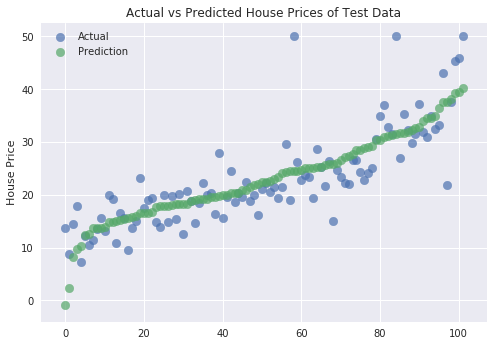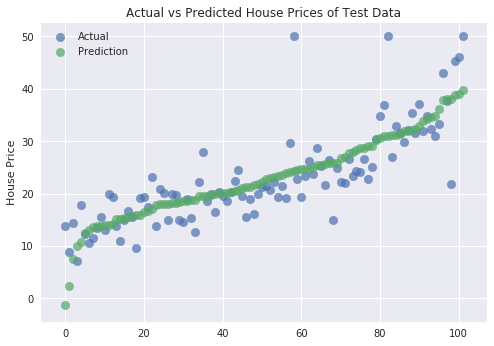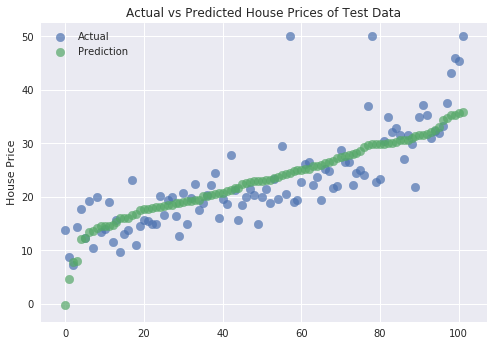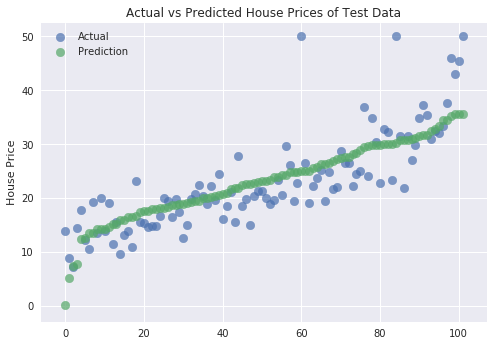# Supervised Learning - Regression¶

Regression is a process where we try to predict a continuous target variable based on independent variables. Scikit-Learn offers various regression models for performing regression learning.

### Applications of Regression¶

• Predicting house price from other attributes like area, no of bedrooms, no of washrooms, parking facility, etc.
• Predicting stock prices based on other attributes.
• Sales of a particular item in the future.
• Temperature prediction
• & many more

### Supervised Learning Workflow¶Let’s use below scikit-learn's various regression models for our purpose.

Scikit-Learn also provides few datasets in-built with a package that we can load directly into memory and use for our purpose. We'll be using one such dataset called the Boston Housing dataset for our purpose. We'll be predicting the house price of a dataset based on other attributes from the dataset.

Below we are starting with importing necessary libraries.

In :
import numpy as np
import pandas as pd

import matplotlib.pyplot as plt

import sklearn

import warnings
import sys

print("Python Version : ",sys.version)
print("Scikit-Learn Version : ",sklearn.__version__)
warnings.filterwarnings("ignore") ## We'll silent future warnings using this command.
np.set_printoptions(precision=3)

## Beow magic function fits plot inside of current notebook.
## There is another option to it (%matplotlib notebook) which opens plot in new notebook.
%matplotlib inline

Python Version :  3.7.3 (default, Mar 27 2019, 22:11:17)
[GCC 7.3.0]
Scikit-Learn Version :  0.21.2


## Linear Regression¶

In the Linear Regression Model, we try to fit the line through data in a way that has a minimum distance from all points in the dataset. Once we have found out proper line which has a minimum distance from all points in data and further optimization is not possible then we use that line to do further prediction on unseen data in the future.

It's also known as Ordinary Least Squares because optimization function tries to minimize the squared distance between the line and all points in Train/Test Set.

We'll load Boston housing data provided by scikit-learn. It returns Bunch object which is almost the same as the dictionary. We'll also print details about the dataset.

In :
from sklearn.datasets import load_boston ## function for loading boston data.
#print(type(boston)) ## It returns Bunch object which is similar to dictionary.
#print(boston.DESCR) ## DESCR attribute describes dataset.
print('Feature Names : ' + str(boston.feature_names))
print('Dataset shape : ' + str(boston.data.shape))
print('Target shape : ' + str(boston.target.shape))

Feature Names : ['CRIM' 'ZN' 'INDUS' 'CHAS' 'NOX' 'RM' 'AGE' 'DIS' 'RAD' 'TAX' 'PTRATIO'
'B' 'LSTAT']
Dataset shape : (506, 13)
Target shape : (506,)


### Splitting Dataset into Train & Test sets¶

We'll split the dataset into two parts:

• Training data which will be used for the training model.
• Test data against which accuracy of the trained model will be checked.

train_test_split function of model_selection module of sklearn will help us split data into two sets with 80% for training and 20% for test purposes. We are also using seed(random_state=123) with train_test_split so that we always get the same split and can reproduce results in the future as well.In :
from sklearn.model_selection import train_test_split # Function for splitting dataset into train/test set.
X = boston.data
Y = boston.target
## We can specify either one of train_size and test_size. Sklearn find out other by itself. I included both for explanation purpose.
## random_state is used to reproduce same data splits again. If we don't set random_state then it generates different splits everytime.
X_train, X_test, Y_train, Y_test = train_test_split(X, Y, train_size=0.8, test_size = 0.2, random_state = 123)
print('Train & Test sizes : ',X_train.shape, X_test.shape, Y_train.shape, Y_test.shape)

Train & Test sizes :  (404, 13) (102, 13) (404,) (102,)


### Initializing Model¶

We are initializing the LinearRegression model below which is the basic model used extensively for regression tasks.

In :
from sklearn.linear_model import LinearRegression ## Linear Regression Implementation
linear_regressor = LinearRegression()
linear_regressor

Out:
LinearRegression(copy_X=True, fit_intercept=True, n_jobs=None, normalize=False)

### Fitting Model To Train Data¶

We can train a model by passing train data and train labels. It returns objects of trained classifier as well after training.

In :
linear_regressor.fit(X_train,Y_train)

Out:
LinearRegression(copy_X=True, fit_intercept=True, n_jobs=None, normalize=False)

### Evaluating Trained Model On Test Data.¶

Almost all models in Scikit-Learn API provides predict() method which can be used to predict the target variables on Test Set passed to it.

We are comparing below housing prices predicted by our model with actual house prices of test data and train data.

In :
y_test_pred = linear_regressor.predict(X_test)
print('First Few Actual Housing Prices(Test Data) : ' + str(Y_test[:5]))
print('First Few Predicted Housing Prices(Test Data) : ' + str(y_test_pred[:5]))

First Few Actual Housing Prices(Test Data) : [15.  26.6 45.4 20.8 34.9]
First Few Predicted Housing Prices(Test Data) : [16.003 27.794 39.268 18.326 30.455]


Scikit-Learn's LinearRegresson model has a score() method which returns coefficient of determination $R^2$ based on the dataset and target variables passed to it. It returns a value between [0-1] with 1 being best. If it returns negative value means that the model performed quite bad.

Note: Do not confuse $R^2$ with MSE as both are quite different. One can calculate MSE by using mean_squared_error provided by the metrics module of sklearn.

Formula of $R^2:$

$R^2 = (1 - u/v)$

where

$u = MSE =((y_{true} - y_{pred})^2).sum()$

$v=((y_{true} - y_{true}.mean())^2).sum()$

In :
print('R^2 Score on Test Data : %.3f'%linear_regressor.score(X_train, Y_train))

R^2 Score on Test Data : 0.756


As we discussed above, linear regression tries to generate lines through data in a way that mean squared error between actual labels and target is least. It is also the reason why its referred to as Ordinary Least Squares by many ML Practitioners as it tries to minimize squared differences between predicted and actual labels. We can access coordinates of that line through coef_ and intercept_ attributes of regressor.

In :
print('Weight Coefficients : '+ str(linear_regressor.coef_))
print('\nY-Axis Intercept : '+ str(linear_regressor.intercept_))

Weight Coefficients : [-9.879e-02  4.750e-02  6.695e-02  1.270e+00 -1.547e+01  4.320e+00
-9.802e-04 -1.366e+00  2.845e-01 -1.275e-02 -9.135e-01  7.226e-03
-5.438e-01]

Y-Axis Intercept : 31.835164121206386


### Visualizing Predictions on Test Data¶

In :
sorted_labels_acc_to_test_y = list(sorted(zip(Y_test, y_test_pred), key=lambda x: x))
sorted_test_y, sorted_test_preds = zip(*sorted_labels_acc_to_test_y)

with plt.style.context(('ggplot', 'seaborn')):
plt.scatter(range(len(sorted_test_y)),sorted_test_y, s=75, alpha=0.7, label='Actual')
plt.scatter(range(len(sorted_test_preds)), sorted_test_preds, s=75, alpha=0.7, label='Prediction')
plt.ylabel('House Price')
plt.title('Actual vs Predicted House Prices of Test Data')
plt.legend(loc='best')## Ridge Regression(L2 Penalty)¶

Ridge regression is another estimator where we introduce regularization(L2 regularization) in the cost minimization function. The introduction of this regularization pushes all weights near zero but not making them exactly zero. It makes all the weight quite small.

### Initializing Model¶

In :
from sklearn.linear_model import Ridge ## Linear Regression Implementation

ridge_regressor = Ridge()
ridge_regressor

Out:
Ridge(alpha=1.0, copy_X=True, fit_intercept=True, max_iter=None,
normalize=False, random_state=None, solver='auto', tol=0.001)

### Fitting Model to Train Data¶

In :
ridge_regressor.fit(X_train,Y_train)

Out:
Ridge(alpha=1.0, copy_X=True, fit_intercept=True, max_iter=None,
normalize=False, random_state=None, solver='auto', tol=0.001)

### Evaluating Trained Model on Test Data¶

In :
y_test_pred = ridge_regressor.predict(X_test)
print('First Few Actual Housing Prices(Test Data) : ' + str(Y_test[:5]))
print('First Few Predicted Housing Prices(Test Data) : ' + str(y_test_pred[:5]))

print('\nR^2 Score on Test Data : %.3f'%ridge_regressor.score(X_test, Y_test))

First Few Actual Housing Prices(Test Data) : [15.  26.6 45.4 20.8 34.9]
First Few Predicted Housing Prices(Test Data) : [15.415 27.674 38.911 17.939 30.564]

R^2 Score on Test Data : 0.650


### Visualizing Predictions on Test Data¶

In :
sorted_labels_acc_to_test_y = list(sorted(zip(Y_test, y_test_pred), key=lambda x: x))
sorted_test_y, sorted_test_preds = zip(*sorted_labels_acc_to_test_y)

with plt.style.context(('ggplot', 'seaborn')):
plt.scatter(range(len(sorted_test_y)),sorted_test_y, s=75, alpha=0.7, label='Actual')
plt.scatter(range(len(sorted_test_preds)), sorted_test_preds, s=75, alpha=0.7, label='Prediction')
plt.ylabel('House Price')
plt.title('Actual vs Predicted House Prices of Test Data')
plt.legend(loc='best')### Finetuning Model By Doing Grid Search On Various Hyperparameters.¶

Below is a list of hyperparameters that we can tune to get the best estimator for our data.

• fit_intercept - It's boolean value referring whether to include intercept in model or not ($y =mx + c$ - here c is referring to intercept).default=True
• alpha - It's regularization strength and helps in reducing overfitting. default=1.0
• solver - Algorithms for optimization. It accepts string from list ['auto', 'svd', 'cholesky', 'lsqr', 'sparse_cg', 'sag', 'saga'] default=auto
• max_iter - It refers to maximum number of iterations for solver to try.default=1000

### GridSearchCV¶

It's a wrapper class provided by sklearn which loops through all parameters provided as params_grid parameter with a number of cross-validation folds provided as cv parameter, evaluates model performance on all combinations and stores all results in cv_results_ attribute. It also stores model which performs best in all cross-validation folds in best_estimator_ attribute and best score in best_score_ attribute.

Note: n_jobs parameter is provided by many estimators. It accepts a number of cores to use for parallelization. If the value of -1 is given then it uses all cores. We are also using %%time which jupyter notebook cell magic command which prints time taken by that cell to complete running. Time will be different on different computers based on their configurations.

In :
%%time

from sklearn.model_selection import GridSearchCV

params = {'alpha' : [500, 200, 100, 50,10, 1, 0.1, 0.01],
'fit_intercept': [True, False],
'solver': ['svd', 'cholesky', 'lsqr', 'sparse_cg', 'sag', 'saga']}

grid = GridSearchCV(Ridge(random_state=1), param_grid=params, cv=3, n_jobs=-1)
grid.fit(X_train, Y_train)

print('Train Accuracy : %.3f'%grid.best_estimator_.score(X_train, Y_train))
print('Test Accuracy : %.3f'%grid.best_estimator_.score(X_test, Y_test))
print('Best Score Through Grid Search : %.3f'%grid.best_score_)
print('Best Parameters : ',grid.best_params_)

Train Accuracy : 0.756
Test Accuracy : 0.658
Best Score Through Grid Search : 0.735
Best Parameters :  {'alpha': 0.1, 'fit_intercept': True, 'solver': 'cholesky'}
CPU times: user 85 ms, sys: 37.5 ms, total: 122 ms
Wall time: 1.58 s


### Printing First Few Cross-Validation Results¶

GridSearchCV object maintains all different parameters tried and results generated for each split of data in an attribute cv_results_ as a dictionary. Below we are loading that cross-validation results as pandas dataframe and printing first few entries.

In :
cross_val_results = pd.DataFrame(grid.cv_results_)
print('Number of Various Combinations of Parameters Tried : %d'%len(cross_val_results))

cross_val_results.head() ## Printing first few results.

Number of Various Combinations of Parameters Tried : 96

Out:
mean_fit_time std_fit_time mean_score_time std_score_time param_alpha param_fit_intercept param_solver params split0_test_score split1_test_score split2_test_score mean_test_score std_test_score rank_test_score
0 0.041272 0.015971 0.000553 0.000027 500 True svd {'alpha': 500, 'fit_intercept': True, 'solver'... 0.701451 0.718088 0.566788 0.662345 0.067660 53
1 0.005146 0.006359 0.000385 0.000081 500 True cholesky {'alpha': 500, 'fit_intercept': True, 'solver'... 0.701451 0.718088 0.566788 0.662345 0.067660 52
2 0.000868 0.000017 0.000349 0.000038 500 True lsqr {'alpha': 500, 'fit_intercept': True, 'solver'... 0.695581 0.709067 0.558561 0.654640 0.067910 56
3 0.001093 0.000124 0.000374 0.000020 500 True sparse_cg {'alpha': 500, 'fit_intercept': True, 'solver'... 0.699746 0.718255 0.558562 0.659102 0.071232 54
4 0.009805 0.001441 0.000390 0.000017 500 True sag {'alpha': 500, 'fit_intercept': True, 'solver'... 0.689287 0.709309 0.545349 0.648236 0.072943 58

## Lasso (L1 Penalty)¶

Lasso Regression is another estimator where we introduce an L1 type of regularization in cost minimization function. L1 type regularization makes few coefficients zero whichever does not have much influence on target variable prediction.

### Initializing Model¶

In :
from sklearn.linear_model import Lasso

lasso_regressor = Lasso()
lasso_regressor

Out:
Lasso(alpha=1.0, copy_X=True, fit_intercept=True, max_iter=1000,
normalize=False, positive=False, precompute=False, random_state=None,
selection='cyclic', tol=0.0001, warm_start=False)

### Fitting Model to Train Data¶

In :
lasso_regressor.fit(X_train,Y_train)

Out:
Lasso(alpha=1.0, copy_X=True, fit_intercept=True, max_iter=1000,
normalize=False, positive=False, precompute=False, random_state=None,
selection='cyclic', tol=0.0001, warm_start=False)

### Evaluating Trained Model on Test Data¶

In :
y_test_pred = lasso_regressor.predict(X_test)
print('First Few Actual Housing Prices(Test Data) : ' + str(Y_test[:5]))
print('First Few Predicted Housing Prices(Test Data) : ' + str(y_test_pred[:5]))

print('\nR^2 Score on Test Data : %.3f'%lasso_regressor.score(X_test, Y_test))

First Few Actual Housing Prices(Test Data) : [15.  26.6 45.4 20.8 34.9]
First Few Predicted Housing Prices(Test Data) : [19.254 27.641 35.712 19.083 29.987]

R^2 Score on Test Data : 0.629


### Visualizing Predictions on Test Data¶

In :
sorted_labels_acc_to_test_y = list(sorted(zip(Y_test, y_test_pred), key=lambda x: x))
sorted_test_y, sorted_test_preds = zip(*sorted_labels_acc_to_test_y)

with plt.style.context(('ggplot', 'seaborn')):
plt.scatter(range(len(sorted_test_y)),sorted_test_y, s=75, alpha=0.7, label='Actual')
plt.scatter(range(len(sorted_test_preds)), sorted_test_preds, s=75, alpha=0.7, label='Prediction')
plt.ylabel('House Price')
plt.title('Actual vs Predicted House Prices of Test Data')
plt.legend(loc='best')### Finetuning Model By Doing Grid Search On Various Hyperparameters.¶

Lasso has exactly the same hyperparameters to tune as that of ridge regression except that it does not have different solver available like ridge.

In :
%%time

from sklearn.model_selection import GridSearchCV

params = {'alpha' : [500, 200, 100, 50,10, 1, 0.1, 0.01],
'fit_intercept': [True, False],
}

grid = GridSearchCV(Lasso(random_state=1), param_grid=params, cv=3, n_jobs=-1)
grid.fit(X_train, Y_train)

print('Train Accuracy : %.3f'%grid.best_estimator_.score(X_train, Y_train))
print('Test Accuracy : %.3f'%grid.best_estimator_.score(X_test, Y_test))
print('Best Score Through Grid Search : %.3f'%grid.best_score_)
print('Best Parameters : ',grid.best_params_)

Train Accuracy : 0.755
Test Accuracy : 0.654
Best Score Through Grid Search : 0.735
Best Parameters :  {'alpha': 0.01, 'fit_intercept': True}
CPU times: user 18.2 ms, sys: 444 µs, total: 18.7 ms
Wall time: 55 ms


### Printing First Few Cross-Validation Results¶

In :
cross_val_results = pd.DataFrame(grid.cv_results_)
print('Number of Various Combinations of Parameters Tried : %d'%len(cross_val_results))

cross_val_results.head() ## Printing first few results.

Number of Various Combinations of Parameters Tried : 16

Out:
mean_fit_time std_fit_time mean_score_time std_score_time param_alpha param_fit_intercept params split0_test_score split1_test_score split2_test_score mean_test_score std_test_score rank_test_score
0 0.000486 0.000061 0.000318 0.000022 500 True {'alpha': 500, 'fit_intercept': True} 0.136585 0.125683 0.138643 0.133625 0.005688 12
1 0.000401 0.000023 0.000326 0.000014 500 False {'alpha': 500, 'fit_intercept': False} -0.014422 0.012669 0.002211 0.000148 0.011169 16
2 0.000456 0.000110 0.000311 0.000022 200 True {'alpha': 200, 'fit_intercept': True} 0.221614 0.249050 0.186873 0.219259 0.025422 11
3 0.000304 0.000038 0.000304 0.000042 200 False {'alpha': 200, 'fit_intercept': False} 0.016237 0.014685 0.025210 0.018695 0.004634 15
4 0.000418 0.000066 0.000366 0.000056 100 True {'alpha': 100, 'fit_intercept': True} 0.233733 0.278236 0.189112 0.233804 0.036362 9

## ElasticNet (L1 & L2 Penalty)¶

ElasticNet is another estimator that uses both L1 and L2 penalty. It's useful in cases where few features are related to one another.

### Initializing Model¶

In :
from sklearn.linear_model import ElasticNet

elasticnet_regressor = ElasticNet()
elasticnet_regressor

Out:
ElasticNet(alpha=1.0, copy_X=True, fit_intercept=True, l1_ratio=0.5,
max_iter=1000, normalize=False, positive=False, precompute=False,
random_state=None, selection='cyclic', tol=0.0001, warm_start=False)

### Fitting Model to Train Data¶

In :
elasticnet_regressor.fit(X_train,Y_train)

Out:
ElasticNet(alpha=1.0, copy_X=True, fit_intercept=True, l1_ratio=0.5,
max_iter=1000, normalize=False, positive=False, precompute=False,
random_state=None, selection='cyclic', tol=0.0001, warm_start=False)

### Evaluating Trained Model on Test Data¶

In :
y_test_pred = elasticnet_regressor.predict(X_test)
print('First Few Actual Housing Prices(Test Data) : ' + str(Y_test[:5]))
print('First Few Predicted Housing Prices(Test Data) : ' + str(y_test_pred[:5]))

print('\nR^2 Score on Test Data : %.3f'%elasticnet_regressor.score(X_test, Y_test))

First Few Actual Housing Prices(Test Data) : [15.  26.6 45.4 20.8 34.9]
First Few Predicted Housing Prices(Test Data) : [19.129 27.548 35.649 19.435 29.83 ]

R^2 Score on Test Data : 0.634


### Visualizing Predictions on Test Data¶

In :
sorted_labels_acc_to_test_y = list(sorted(zip(Y_test, y_test_pred), key=lambda x: x))
sorted_test_y, sorted_test_preds = zip(*sorted_labels_acc_to_test_y)

with plt.style.context(('ggplot', 'seaborn')):
plt.scatter(range(len(sorted_test_y)),sorted_test_y, s=75, alpha=0.7, label='Actual')
plt.scatter(range(len(sorted_test_preds)), sorted_test_preds, s=75, alpha=0.7, label='Prediction')
plt.ylabel('House Price')
plt.title('Actual vs Predicted House Prices of Test Data')
plt.legend(loc='best')### Finetuning Model By Doing Grid Search On Various Hyperparameters.¶

ElasticNet has all parameters the same as that of Ridge and Lasso with one extra parameter which maintains the proportion of L1 and L2 penalty to be used in the regression model.

• l1_ratio - It's float value between [0,1] for controlling proportion of L1 and L2 penalties. The Value of 0 refers to the L2 penalty and the value of 1 refers to the L1 penalty. All in-between values refers to combinations of both L1 and L2. default=0.5
In :
%%time

from sklearn.model_selection import GridSearchCV

params = {'alpha' : [500, 200, 100, 50,10, 1, 0.1, 0.01],
'fit_intercept': [True, False],
'l1_ratio': [0,0.3, 0.5, 0.7, 1.0]
}

grid = GridSearchCV(ElasticNet(random_state=1), param_grid=params, cv=3, n_jobs=-1)
grid.fit(X_train, Y_train)

print('Train Accuracy : %.3f'%grid.best_estimator_.score(X_train, Y_train))
print('Test Accuracy : %.3f'%grid.best_estimator_.score(X_test, Y_test))
print('Best Score Through Grid Search : %.3f'%grid.best_score_)
print('Best Parameters : ',grid.best_params_)

Train Accuracy : 0.755
Test Accuracy : 0.654
Best Score Through Grid Search : 0.735
Best Parameters :  {'alpha': 0.01, 'fit_intercept': True, 'l1_ratio': 1.0}
CPU times: user 50.2 ms, sys: 0 ns, total: 50.2 ms
Wall time: 156 ms


### Printing First Few Cross-Validation Results¶

In :
cross_val_results = pd.DataFrame(grid.cv_results_)
print('Number of Various Combinations of Parameters Tried : %d'%len(cross_val_results))

cross_val_results.head() ## Printing first few results.

Number of Various Combinations of Parameters Tried : 80

Out:
mean_fit_time std_fit_time mean_score_time std_score_time param_alpha param_fit_intercept param_l1_ratio params split0_test_score split1_test_score split2_test_score mean_test_score std_test_score rank_test_score
0 0.062457 6.679862e-04 0.000407 0.000005 500 True 0 {'alpha': 500, 'fit_intercept': True, 'l1_rati... 0.327428 0.370264 0.240512 0.312913 0.053927 42
1 0.000347 4.031664e-05 0.000286 0.000012 500 True 0.3 {'alpha': 500, 'fit_intercept': True, 'l1_rati... 0.227756 0.261245 0.186892 0.225393 0.030382 56
2 0.000339 2.304110e-05 0.000289 0.000018 500 True 0.5 {'alpha': 500, 'fit_intercept': True, 'l1_rati... 0.211594 0.232031 0.184351 0.209387 0.019516 59
3 0.000275 1.486801e-06 0.000264 0.000002 500 True 0.7 {'alpha': 500, 'fit_intercept': True, 'l1_rati... 0.187604 0.195379 0.172910 0.185328 0.009308 61
4 0.000270 7.867412e-07 0.000261 0.000002 500 True 1 {'alpha': 500, 'fit_intercept': True, 'l1_rati... 0.136585 0.125683 0.138643 0.133625 0.005688 63

Please make a note of $R^2$ calculated by each model on the train and test data. Ridge performs better than Linear Regression. Lasso Performs better than Ridge and Linear Regression. Elastic Net seems to perform almost the same as Lasso or little better than it. One can try these models on various datasets to check the performance of each one.Sunny Solanki